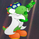צפיות ‎5260‎
5260
This is a T3 CCI with a fast and slow line as well as extreme lines, a -15,15 filter to make zero line rejections and crosses more mechanical and help weed out whipsaw. I will probably update description in the future and get into more detail about how the indicator is used but for now if you want more info look up woodie CCI patterns :) Good Luck!!
```//@version=2
study(title="Trend and Entry CCI ST15", shorttitle="Trend and Entry CCI")
CCI_Period = input(89, minval=1)
CCI_Period14 = input(21, minval=2)
T3_Period = input(5, minval=1)
b = input(0.618)
band5 = hline(100)
band4 = hline(-100)
hline(0, color=purple, linestyle=line)
band3 = hline(200, title="Upper Line", linestyle=dashed, linewidth=2, color=red)
band2 = hline(-200, title="Lower Line", linestyle=dashed, linewidth=2, color=lime)
band1 = hline(15)
band0 = hline(-15)
fill(band1, band0, color=yellow, transp=1)
xPrice = close
b2 = b*b
b3 = b2*b
c1 = -b3
c2 = (3*(b2 + b3))
c3 = -3*(2*b2 + b + b3)
c4 = (1 + 3*b + b3 + 3*b2)
nn = iff(T3_Period < 1, 1, T3_Period)
nr = 1 + 0.5*(nn - 1)
w1 = 2 / (nr + 1)
w2 = 1 - w1
xcci = cci(xPrice, CCI_Period)
e1 = w1*xcci + w2*nz(e1)
e2 = w1*e1 + w2*nz(e2)
e3 = w1*e2 + w2*nz(e3)
e4 = w1*e3 + w2*nz(e4)
e5 = w1*e4 + w2*nz(e5)
e6 = w1*e5 + w2*nz(e6)
xccir = c1*e6 + c2*e5 + c3*e4 + c4*e3
cciHcolor = iff(xccir >= 0 , green,iff(xccir < 0, red, black))

xcci14 = cci(xPrice, CCI_Period14)
a1 = w1*xcci14 + w2*nz(a1)
a2 = w1*a1 + w2*nz(a2)
a3 = w1*a2 + w2*nz(a3)
a4 = w1*a3 + w2*nz(a4)
a5 = w1*a4 + w2*nz(a5)
a6 = w1*a5 + w2*nz(a6)
xccir14 = c1*a6 + c2*a5 + c3*a4 + c4*a3

plot(xccir, color=blue, title="T3-CCI")
plot(xccir, color=cciHcolor, title="CCIH", style = histogram)

plot(xccir14, color=orange, title="CCIH",linewidth=2, style = line)```I think a great way to use this indicator as a stand alone system is enter when you get a trendline break of the slow CCI line and exit when the fast line crosses under -100 if you are in a long position or over 100 if you are in a short position.
השבYou are welcome guys. My favorite way to use it is to enter with trendline breaks on the histogram (slow line). They T3EE indicator i made compliments it this indicator very well.
השב